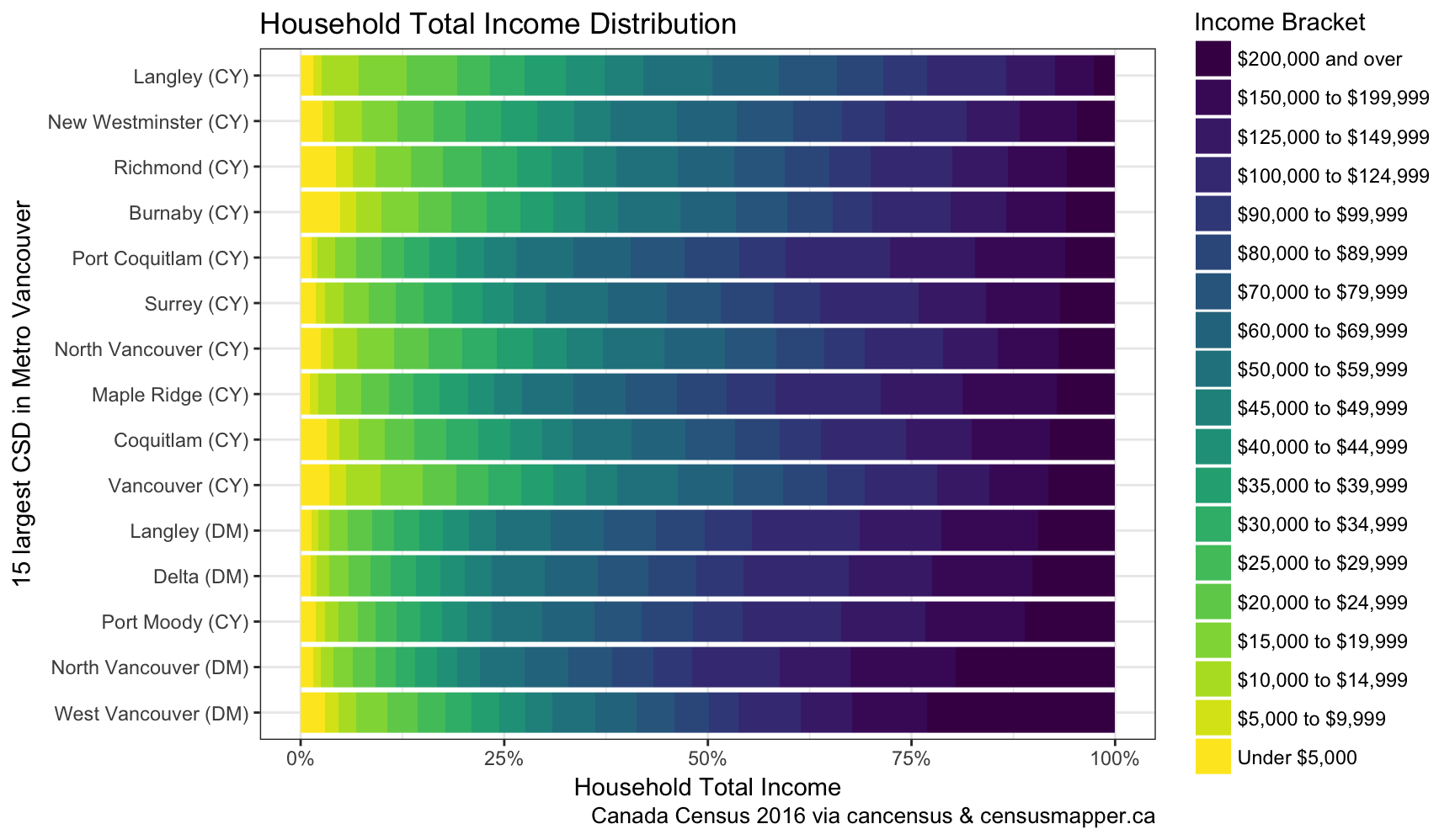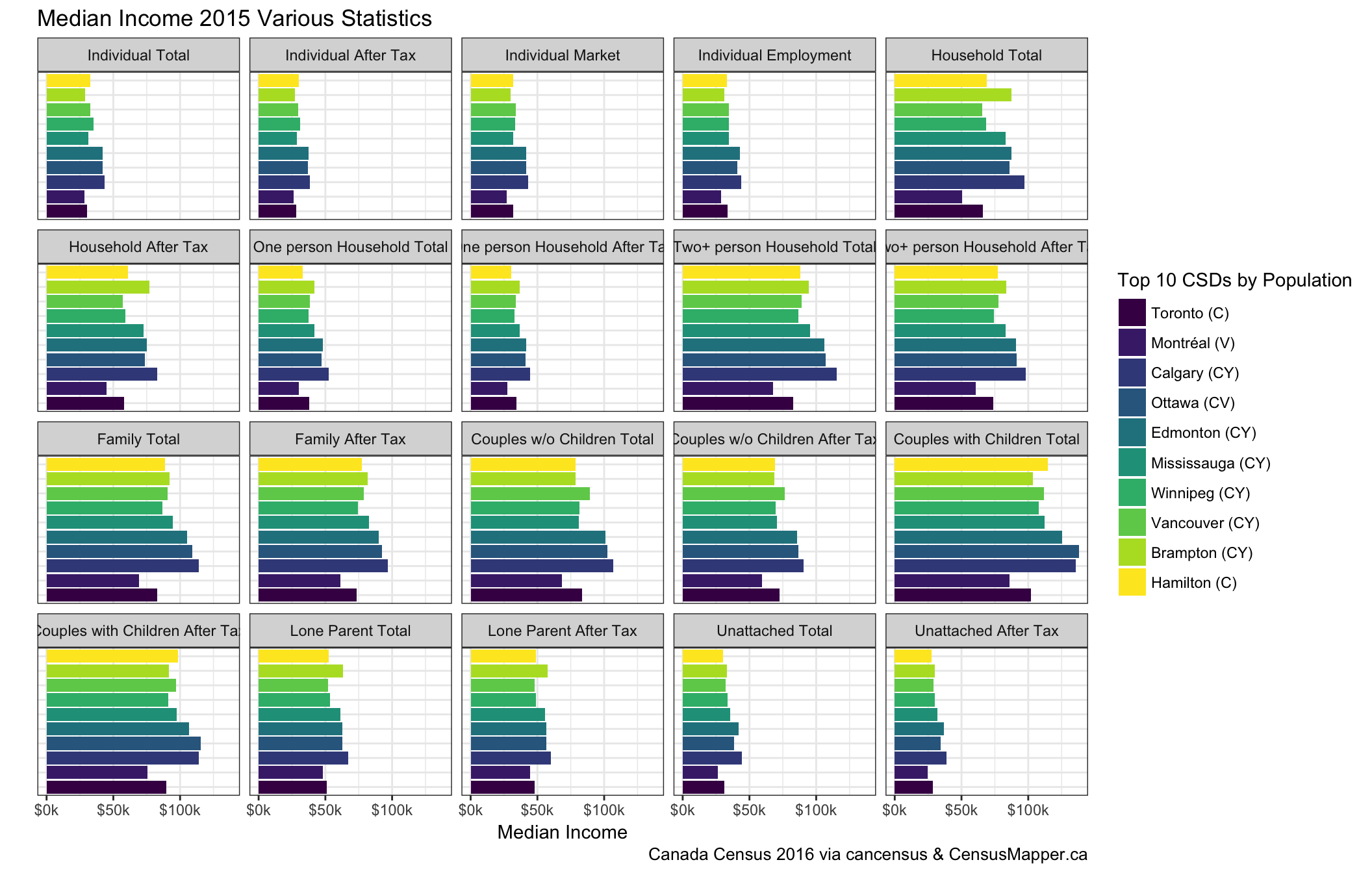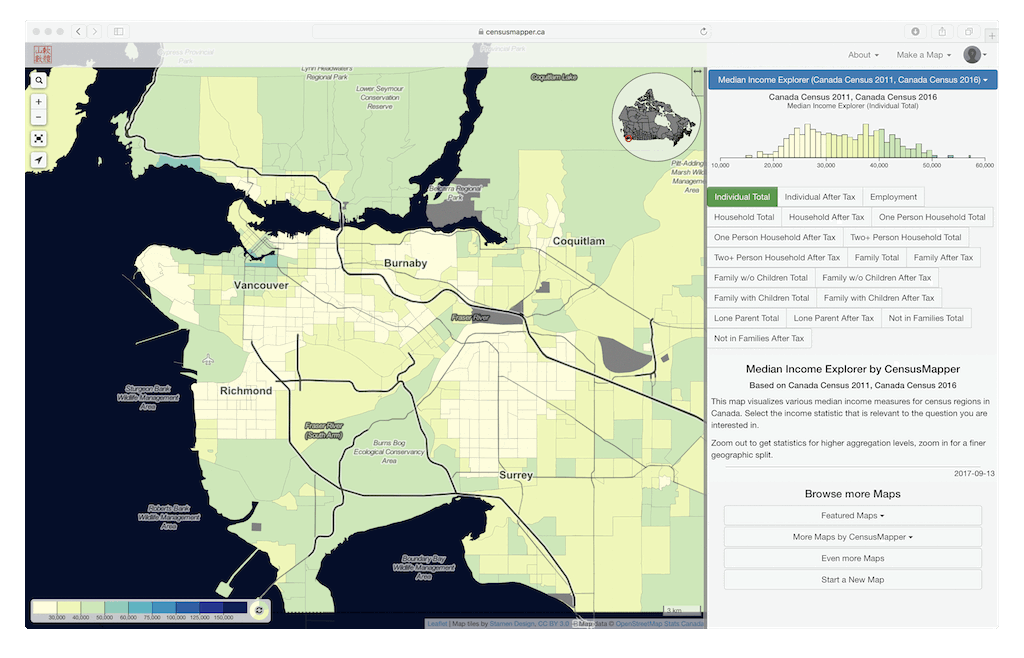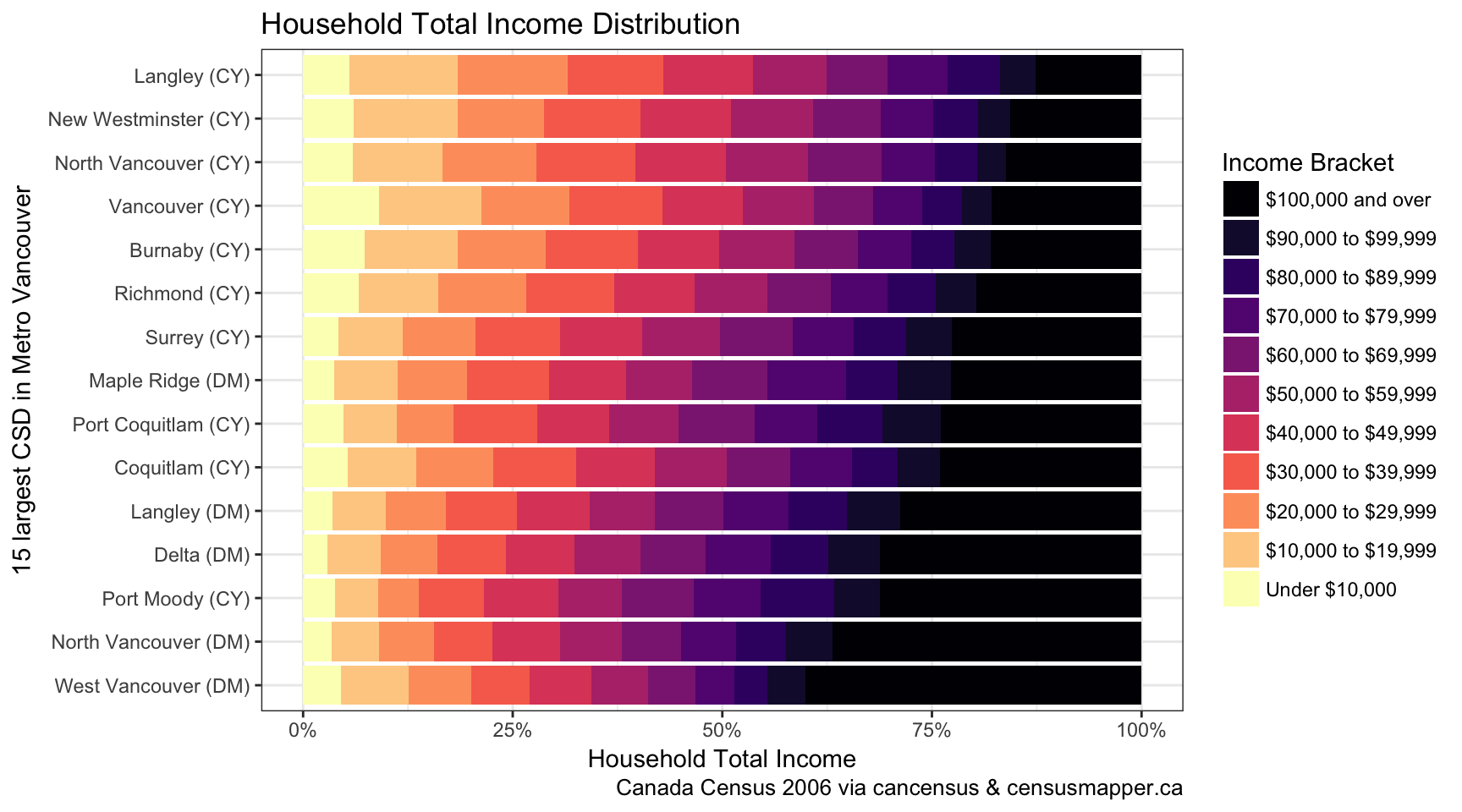# Income - A First Look

Incomes are complex.Income numbers for the 2016 census are out, and I am taking a first shot to dig a little into the numbers. The numbers correspond to the 2015 income tax data, and this census was the first time that all data was directly linked to CRA tax data. For all people. So the income data is part of the “100% data” this time.

In the standard release we got median income data (no average income numbers this year, for better or worse), individual and household income distributions, income deciles for families, and two low income measures by rough age groups. That’s a lot to digest. I have made some graphs to make sense of it, and got nudged to share my code. That’s a good idea, so I packed it into a blog post. For readability I hide some of the code, but the full R notebook lives on GitHub.

## Median Incomes

The first look is usually at median incomes, it breaks things down into one simple, easily digestible number. The only trouble is, what the median for which income statistic should we use? Let’s get an overview of what there is.

Let’s load in some census data to see. As an example, let’s look at income statistics for the 10 most populous cities.

#devtools::install_github("mountainmath/cancensus")
library(cancensus)
dataset='CA16'
level="CSD"
median_income_vectors <- list_census_vectors(dataset, quiet=TRUE) %>%
filter(type=="Total",grepl("Median",label),grepl("income",label)) %>% pull("vector")
regions <- list_census_regions(dataset) %>% filter(level==!!level) %>% top_n(10,pop) %>% as_census_region_list


data <- get_census(dataset = 'CA16',
level="Regions",
vectors=median_income_vectors ,
regions=regions,
geo_format = NA,
labels='short')


and graphing it gives

plot_data <- data %>% select(c("Region Name",median_income_vectors)) %>%
reshape2::melt(id="Region Name") %>%
mutate(Region Name = factor(Region Name,
levels = data %>% arrange(desc(Population)) %>% pull("Region Name"),
ordered=TRUE))
plot_data$var <- factor(as.character(labels[plot_data$variable]),levels=as.character(labels),ordered=TRUE)

ggplot(plot_data , aes(x = Region Name, y = value, fill=Region Name)) +
geom_bar(stat = "identity") +
facet_wrap(~var) +
scale_y_continuous(labels=currency_format_short) +
labs(fill = paste0("Top 10 ",level,"s by Population"),
y = "Median Income",
x="",
title="Median Income 2015 Various Statistics",
caption="Canada Census 2016 via cancensus & CensusMapper.ca") +
theme_bw() +
theme(axis.ticks.y=element_blank(),axis.text.y=element_blank()) +
coord_flip()Overall, the statistics look quite similar, but details matter. Comparing incomes in Toronto and Vancouver we see that while Toronto’s median income at $65,829 is higher than Vancouver’s$65,327, that’s entirely due to the different household composition of these two cities, Vancouver has proportionally more one-person households. Splitting things up we can look at median one-person household income, where Vancouver’s $38,449 edges out Toronto’s$38,018, and at median two-or-more-person household income, where Vancouver’s $89,207 easily beats Toronto’s$82,908.

Bottom line, it matters how we boil down the numbers. On CensusMapper we built and easy way to explore the various median income statistics further.

## Income Distributions

Let’s go beyond the medians and look at distributions. Let’s compare how the top 15 municipalities in Metro Vancouver stack up. We will hide code (it is on GitHub] if you are interested in playing with it), but simply grab the relevant data and graph it.

graph_distributions_for_year(2016)## Income Through Time

The same code that visualized the income distribution for 2015 data works for other census years too (with some mild case checking), we we can easily visualize the 2005 data (from the 2016 census).

graph_distributions_for_year(2006)But doing actual comparisons through time is much harder. Firstly, the income brackets don’t line up. That’s not that big a deal though, one could re-group the data. But depending what we want to use the data for we probably want to adjust for inflation. And that messes up the bracket cutoffs anyway, and we need to become creative.

There are plenty of ways to mess this up, some media outlets have adjusted twice for inflation, others have compared inflation adjusted income gains against the (by definition) unadjusted HPI. We will save diving into comparing incomes across time for another post. And maybe also take a look how 2010 NHS income data fits in between 2005 and 2015, there has been lots of hand-wringing about that data and I am quite curious how the extensive StatCan post-processing of NHS income data stacks up.